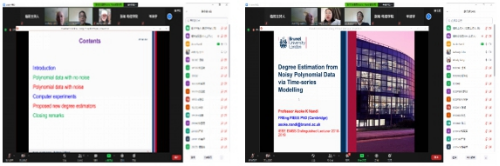﻿ "Degree Estimation from Noisy Polynomial Data Based on Time Series Modelling" by Professor A K Nandi-College of Electronic and Information EngineeringHome
Home   >   News&Events   >   Content

# "Degree Estimation from Noisy Polynomial Data Based on Time Series Modelling" by Professor A K Nandi

Time:May 8, 2021          Browse:

On April 22, 2021, the 5th Zhi·Xin lecture hall of our college was held in the form of online ZOOM conference. Professor Asoke Nandi from Brunel University London was invited to give a lecture.

In recent years, the theory and algorithm of degree estimation are more and more mature, and the application fieldsof it are more and more wide. In many practical applications, accurately estimating the degree of polynomials from data can significantly improve the performance in modeling, detection, estimation, and prediction. Therefore, accurate degree estimation is particularly important in data science.

Professor Asoke Nandi's main research is to use only one unknown to represent any degree polynomial of the same order time series. He took "Degree Estimation of Noisy Polynomial Data Based on Time Series Modelling" as the lecture title, mainly analyzed the characteristics of polynomial data from the aspects of noisy polynomial, noisy polynomial, computer experiment, new degree estimation and put forward a new method to derive degree estimation from unknown parameters. Firstly, first order, second order polynomials and autoregressive time serieswere introduced, and a new degree estimation method of noisy polynomials was proposed. Thenhe explained the number of unknown parameters of the noiseless time series equation and the polynomial equation, and elucidated the relationship between the number of unknown parameters of the polynomial with noise and the noise value, and proposed that the mean square error decreases with the increase of the degree of the fitting polynomial and the change of other parameters. The theory, process and results of computer experiments were described in detail, and the corresponding relationship of degree estimation to each noise standard deviation was pointed out. Finally, the polynomial was represented by a time series model which contains only one unknown constant of known form, and the degree of polynomial was accurately estimated by the time series model without using any polynomial coefficients, and the veracity of some parameters was compared.

It is an important goal of our university to excavate data in depth and accurately and apply it more widely. In the future, our Tongji university will continue to conduct in-depth exchanges and cooperation with Brunel University London in the UK.Written by Gong Xiaoliang

Photographed by Gong Xiaoliang

CLOSE